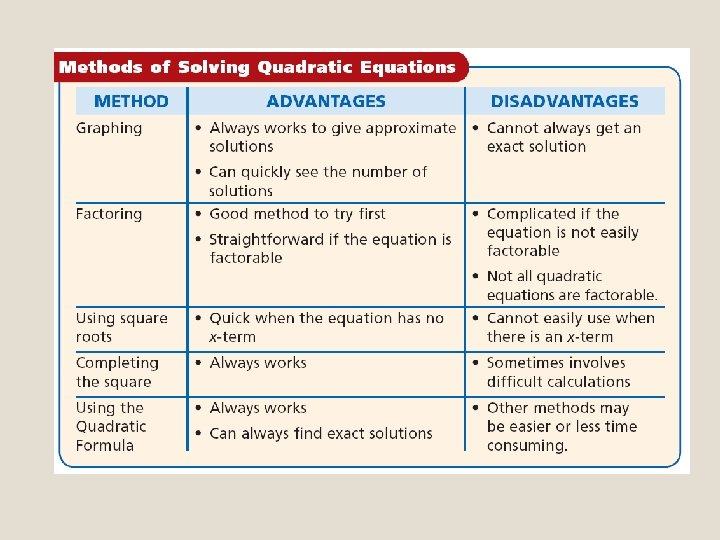# Algebra 1 B Chapter 9 Solving Quadratic Equations

• Slides: 29Algebra 1 B Chapter 9 Solving Quadratic Equations The Quadratic Formula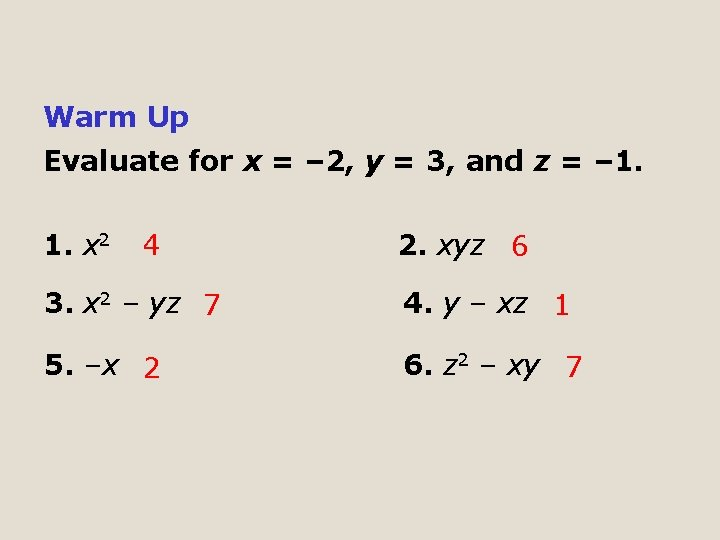Warm Up Evaluate for x = – 2, y = 3, and z = – 1. 1. x 2 4 2. xyz 6 3. x 2 – yz 7 4. y – xz 1 5. –x 2 6. z 2 – xy 7In the previous lesson, you completed the square to solve quadratic equations. If you complete the square of ax 2 + bx + c = 0, you can derive the Quadratic Formula.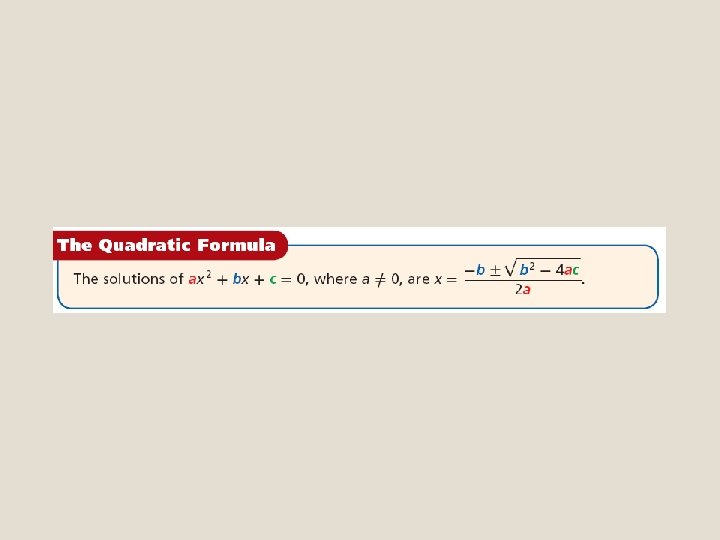Remember! To add fractions, you need a common denominator.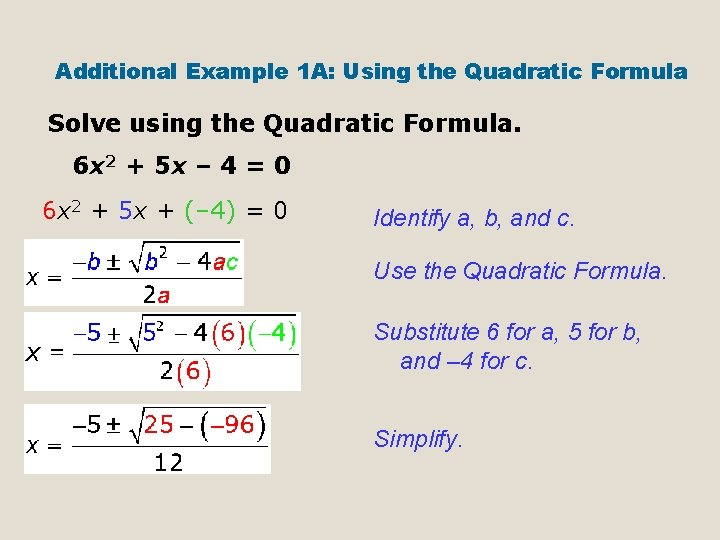Additional Example 1 A: Using the Quadratic Formula Solve using the Quadratic Formula. 6 x 2 + 5 x – 4 = 0 6 x 2 + 5 x + (– 4) = 0 Identify a, b, and c. Use the Quadratic Formula. Substitute 6 for a, 5 for b, and – 4 for c. Simplify.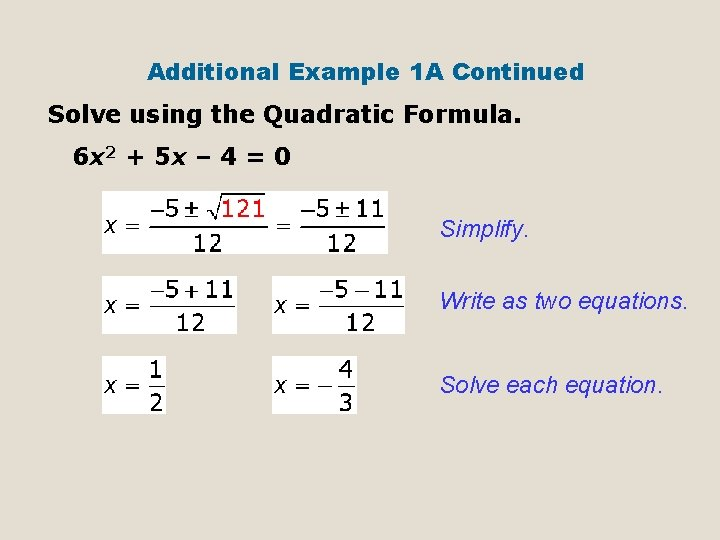Additional Example 1 A Continued Solve using the Quadratic Formula. 6 x 2 + 5 x – 4 = 0 Simplify. Write as two equations. Solve each equation.Additional Example 1 B: Using the Quadratic Formula Solve using the Quadratic Formula. x 2 = x + 20 1 x 2 + (– 1 x) + (– 20) = 0 Write in standard form. Identify a, b, and c. Use the Quadratic Formula. Substitute 1 for a, – 1 for b, and – 20 for c. Simplify.Additional Example 1 B Continued Solve using the Quadratic Formula. x 2 = x + 20 Simplify. Write as two equations. x=5 or x = – 4 Solve each equation.In Your Notes! Example 1 a Solve using the Quadratic Formula. Check your answer. – 3 x 2 + 5 x + 2 = 0 Identify a, b, and c. Use the Quadratic Formula. Substitute – 3 for a, 5 for b, and 2 for c. Simplify.In Your Notes! Example 1 a Continued Solve using the Quadratic Formula. Check your answer. – 3 x 2 + 5 x + 2 = 0 Simplify. Write as two equations. x=– or x=2 Solve each equation.In Your Notes! Example 1 b Solve using the Quadratic Formula. Check your answer. 2 – 5 x 2 = – 9 x (– 5)x 2 + 9 x + (2) = 0 Write in standard form. Identify a, b, and c. Use the Quadratic Formula. Substitute – 5 for a, 9 for b, and 2 for c. Simplify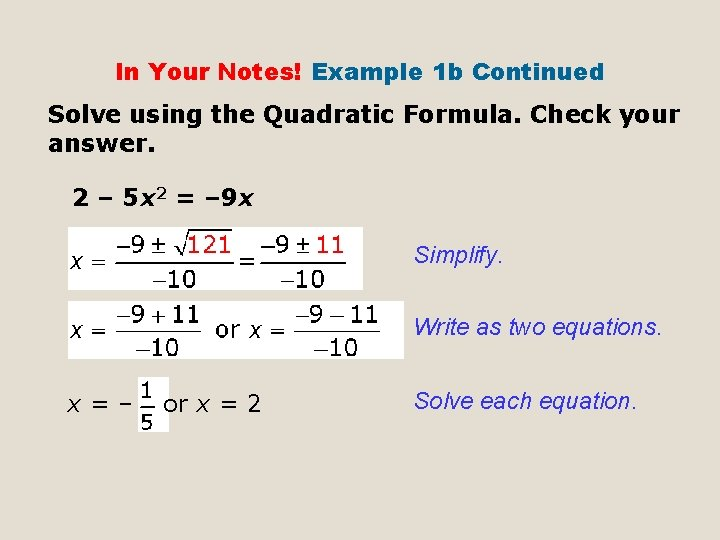In Your Notes! Example 1 b Continued Solve using the Quadratic Formula. Check your answer. 2 – 5 x 2 = – 9 x Simplify. Write as two equations. x=– or x = 2 Solve each equation.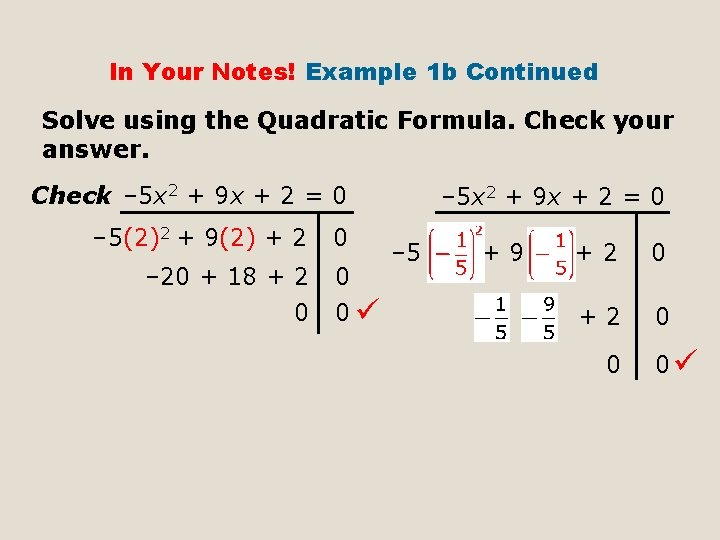In Your Notes! Example 1 b Continued Solve using the Quadratic Formula. Check your answer. Check – 5 x 2 + 9 x + 2 = 0 – 5(2)2 + 9(2) + 2 – 20 + 18 + 2 0 0 – 5 x 2 + 9 x + 2 = 0 – 5 +9 +2 0 0 0Because the Quadratic Formula contains a square root, the solutions may be irrational. You can give the exact solution by leaving the square root in your answer, or you can approximate the solutions.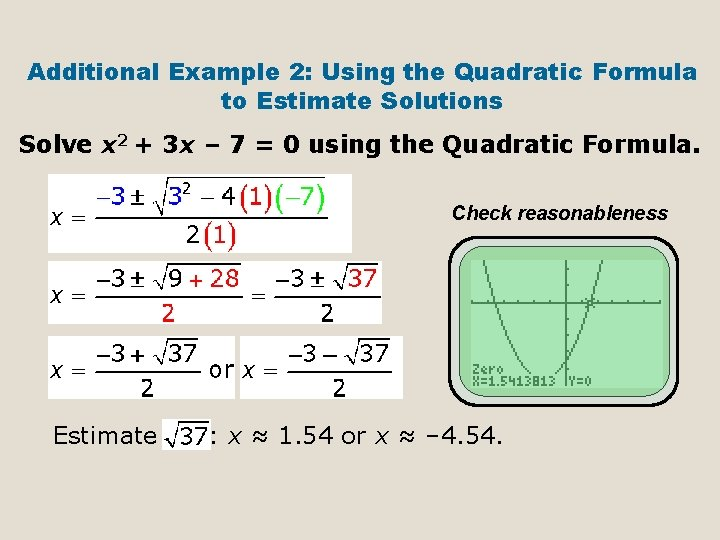Additional Example 2: Using the Quadratic Formula to Estimate Solutions Solve x 2 + 3 x – 7 = 0 using the Quadratic Formula. Check reasonableness Estimate : x ≈ 1. 54 or x ≈ – 4. 54.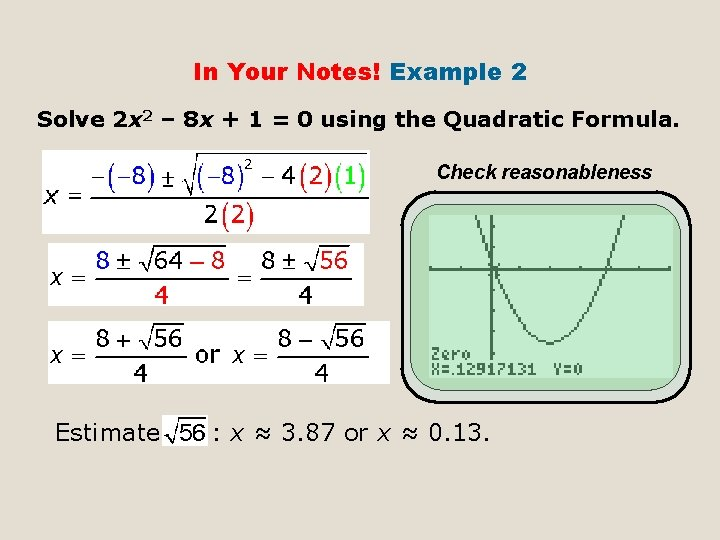In Your Notes! Example 2 Solve 2 x 2 – 8 x + 1 = 0 using the Quadratic Formula. Check reasonableness Estimate : x ≈ 3. 87 or x ≈ 0. 13.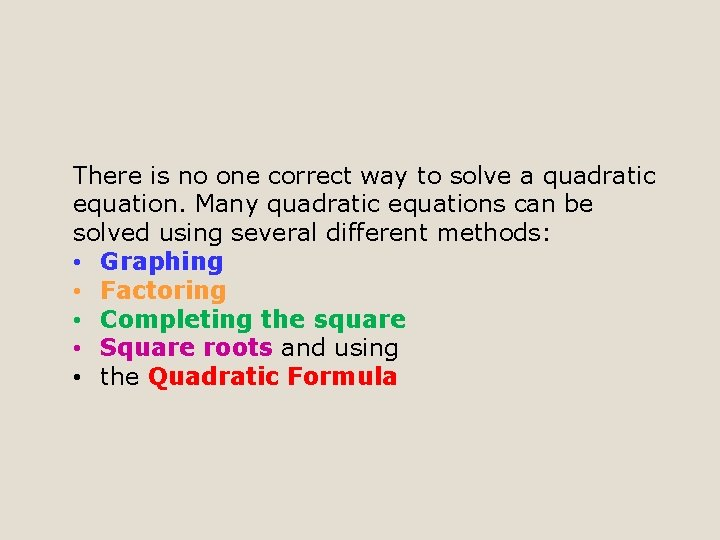There is no one correct way to solve a quadratic equation. Many quadratic equations can be solved using several different methods: • Graphing • Factoring • Completing the square • Square roots and using • the Quadratic Formula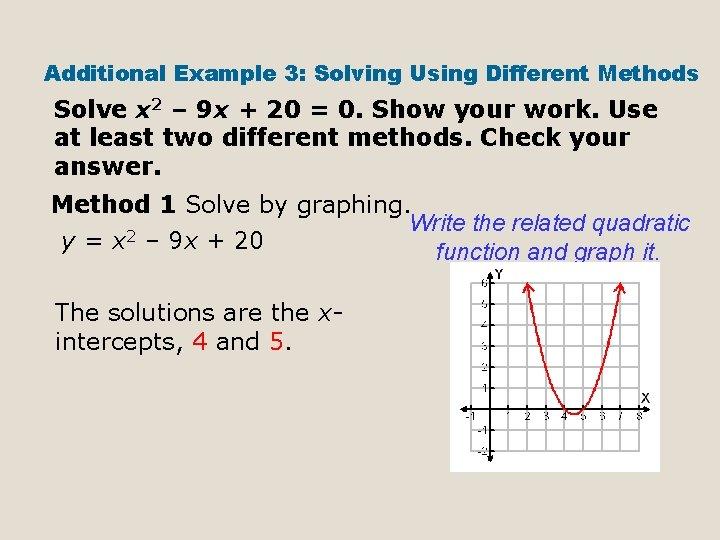Additional Example 3: Solving Using Different Methods Solve x 2 – 9 x + 20 = 0. Show your work. Use at least two different methods. Check your answer. Method 1 Solve by graphing. Write the related quadratic 2 y = x – 9 x + 20 function and graph it. The solutions are the xintercepts, 4 and 5.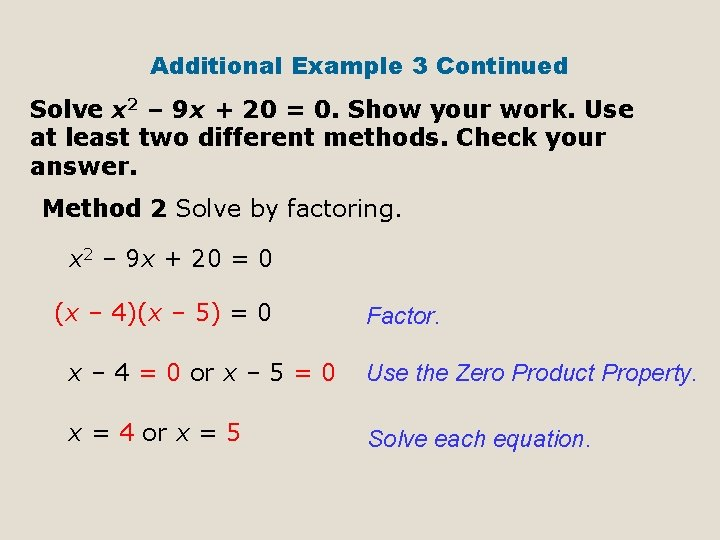Additional Example 3 Continued Solve x 2 – 9 x + 20 = 0. Show your work. Use at least two different methods. Check your answer. Method 2 Solve by factoring. x 2 – 9 x + 20 = 0 (x – 4)(x – 5) = 0 Factor. x – 4 = 0 or x – 5 = 0 Use the Zero Product Property. x = 4 or x = 5 Solve each equation.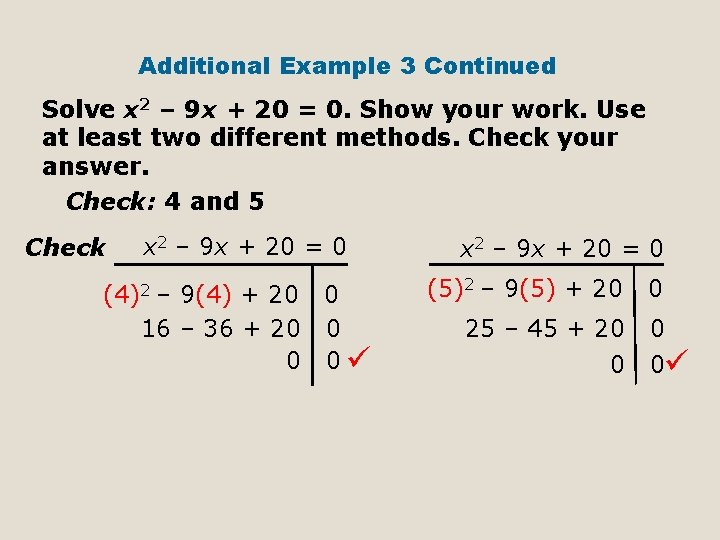Additional Example 3 Continued Solve x 2 – 9 x + 20 = 0. Show your work. Use at least two different methods. Check your answer. Check: 4 and 5 Check x 2 – 9 x + 20 = 0 (4)2 – 9(4) + 20 0 16 – 36 + 20 0 x 2 – 9 x + 20 = 0 (5)2 – 9(5) + 20 25 – 45 + 20 0 0In Your Notes! Example 3 a Solve. Show your work and check your answer. x 2 + 7 x + 10 = 0 Method 3 Solve by completing the square. x 2 + 7 x + 10 = 0 x 2 + 7 x = – 10 x 2 +7 x = – 10 Add to both sides. Factor and simplify. Take the square root of both sides.In Your Notes! Example 3 a Continued Solve. Show your work and check your answer. x 2 + 7 x + 10 = 0 or Solve each equation. x = – 2 or x = – 5 Check x 2 + 7 x + 10 = 0 (– 2)2 + 7(– 2) + 10 4 – 14 + 10 0 0 x 2 + 7 x + 10 = 0 (– 5)2 + 7(– 5) + 10 0 25 – 35 + 10 0In Your Notes! Example 3 b Solve. Show your work and check your answer. – 14 + x 2 – 5 x = 0 Method 4 Solve using the Quadratic Formula. x 2 – 5 x – 14 = 0 1 x 2 – 5 x – 14 = 0 Identify a, b, and c. Substitute 1 for a, – 5 for b, and – 14 for c. Simplify.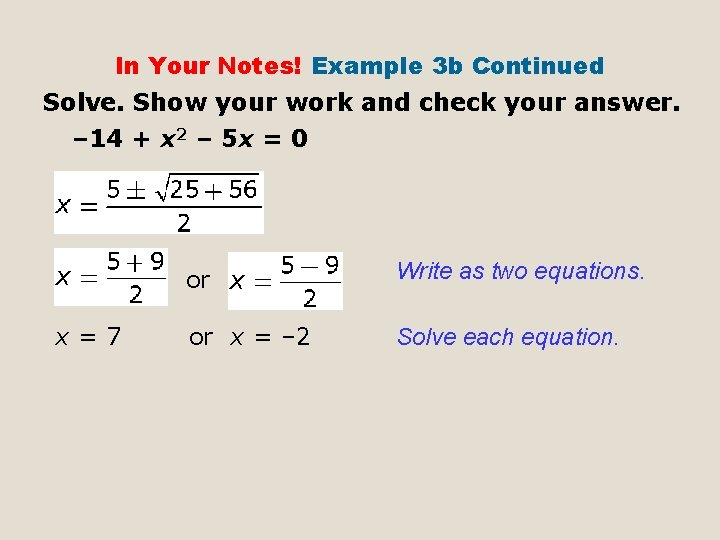In Your Notes! Example 3 b Continued Solve. Show your work and check your answer. – 14 + x 2 – 5 x = 0 x=7 or Write as two equations. or x = – 2 Solve each equation.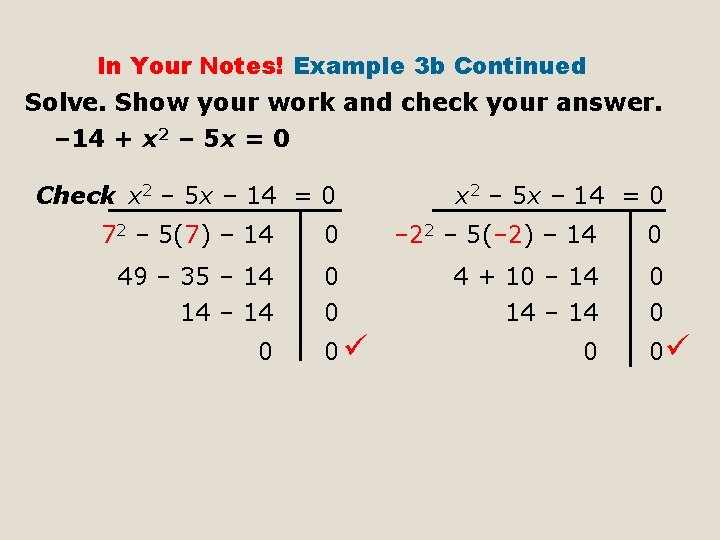In Your Notes! Example 3 b Continued Solve. Show your work and check your answer. – 14 + x 2 – 5 x = 0 Check x 2 – 5 x – 14 = 0 72 – 5(7) – 14 0 – 22 – 5(– 2) – 14 0 49 – 35 – 14 0 4 + 10 – 14 0 14 – 14 0 0 0In Your Notes! Example 3 c Solve. Show your work and check your answer. 2 x 2 + 4 x – 21 = 0 Method 1 Solve by graphing. Write the related quadratic 2 x 2 + 4 x – 21 = y function. Divide each term by 2 and graph. The solutions are the x-intercepts and appear to be ≈ 2. 4 and ≈ – 4. 4.Sometimes one method is better for solving certain types of equations. The table below gives some advantages and disadvantages of the different methods.